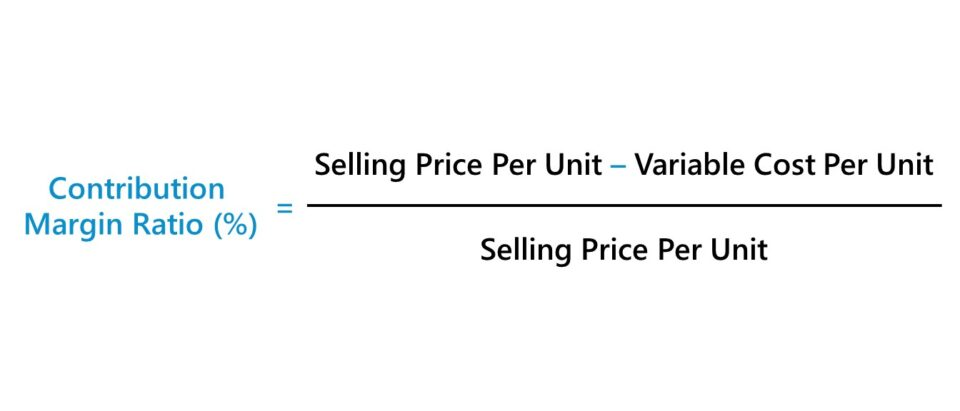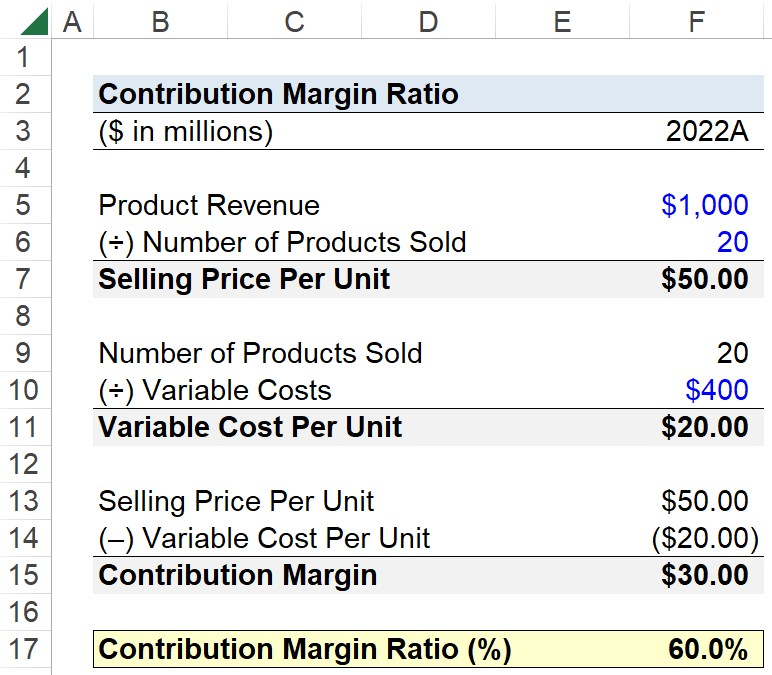Welcome to Wall Street Prep! Use code at checkout for 15% off.# Contribution Margin Ratio

Guide to Understanding the Contribution Margin Ratio (CM)## How to Calculate Contribution Margin Ratio?

The contribution margin ratio, often abbreviated as “CM ratio”, expresses the residual profits generated from each unit of product sold, once all variable costs are subtracted from product revenue.

The difference between variable costs and fixed costs is as follows.

• Variable Costs (VC) → The incurred costs directly tied to and fluctuate based upon production volume (i.e. output activity) in the current period. Based on the production output in the given period, a company’s variable costs – such as direct labor and raw material costs – fluctuate either upward or downward, since such costs support the generation of revenue.
• Fixed Costs (FC) → These incurred costs remain relatively the same, regardless of the company’s production volume in the given period. Fixed costs are independent of production volume and are incurred regardless of the circumstances. For instance, a company’s rental expense on an office space must be paid each month, whether its sales are thriving or if the company is on the verge of bankruptcy.

The calculation of the contribution margin ratio is a three-step process.

1. Calculate the Contribution Margin (Selling Price Per Unit – Variable Cost Per Unit)
2. Divide the Contribution Margin by the Selling Price Per Unit
3. Multiply the Resulting Ratio by 100 to Convert into Percentage Form

## Contribution Margin Ratio Formula

The formula to calculate the contribution margin ratio (or CM ratio) is as follows.

Contribution Margin Ratio (%) = (Selling Price Per Unit  Variable Cost Per Unit) ÷ Selling Price Per Unit

The resulting ratio compares the contribution margin per unit to the selling price of each unit to understand the specific costs of a particular product.

Since the incremental profit earned on each product sale can be derived from the ratio, the ratio offers useful information that can help guide decisions, such as setting product pricing appropriately to ensure a minimum margin is met (and the business model is sustainable).

## Contribution Margin Ratio Calculator

We’ll now move on to a modeling exercise, which you can access by filling out the form below.Submitting...

## Contribution Margin Ratio Calculation Example

Suppose you’re tasked with calculating the contribution margin ratio of a company’s product.

In 2022, the product generated \$1 billion in revenue, with 20 million units sold, alongside \$400 million in variable costs.

• Product Revenue = \$1 billion
• Number of Products Sold = 20 million
• Variable Costs = \$400 million

The product revenue and number of products sold can be divided to determine the selling price per unit, which is \$50.00 per product.

•   Selling Price Per Unit = \$1 billion ÷ \$20 million = \$50.00

In the next part, we must calculate the variable cost per unit, which we’ll determine by dividing the total number of products sold by the total variable costs incurred.

• Variable Cost Per Unit = \$400 million ÷ 20 million = \$20.00

Only two more steps remain in our quick exercise, starting with the calculation of the contribution margin per unit – the difference between the selling price per unit and variable cost per unit – which equals \$30.00.

• Contribution Margin = \$50.00 – \$20.00 = \$30.00

The \$30.00 represents the earnings remaining after deducting variable costs (and is left over to cover fixed costs and more).

In conclusion, we’ll calculate the product’s contribution margin ratio (%) by dividing its contribution margin per unit by its selling price per unit, which returns a ratio of 0.60, or 60%.

• Contribution Margin Ratio (%) = \$30.00 ÷ \$50.00 = 60.0%Step-by-Step Online Course

### Everything You Need To Master Financial Modeling

Enroll in The Premium Package: Learn Financial Statement Modeling, DCF, M&A, LBO and Comps. The same training program used at top investment banks.